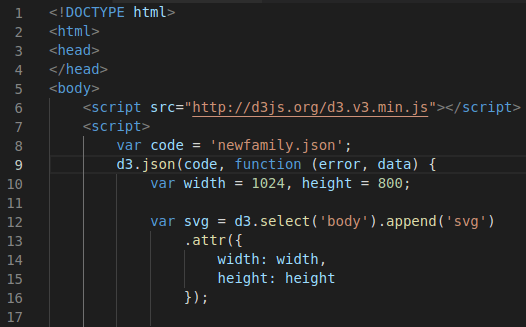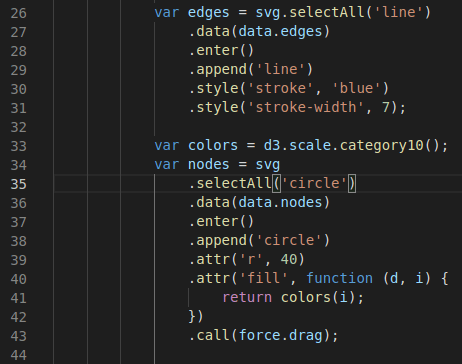# Force-Directed Graph with D3.js

Network visualizations involve displaying interconnected nodes commonly associated with social networks. D3.js has powerful capabilities to create these visualizations. In this post, we will learn how to make a simple force-directed graph.

A force directed graph uses an algorithm that spaces the nodes in the graph away from each other based on a value you set. There are several different ways to determine how the force influences the distance of the nodes from each other that will be explored somewhat in this post.The Data

To make the visualization it is necessary to have data. We will use a simple json file that has nodes and edges. Below is the code

```{

"nodes": [

{ "name": "Tom" },

{ "name": "Sue" },

{ "name": "Jina" },

{ "name": "Soli" },

{ "name": "Lala" }

],

"edges": [

{ "source": 0, "target": 1 },

{ "source": 0, "target": 4 },

{ "source": 0, "target": 3 },

{ "source": 0, "target": 4 },

{ "source": 0, "target": 2 },

{ "source": 1, "target": 2 }

]

}```

The nodes in this situation will represent the circles that we will make. In this case, the nodes have names. However, we will not print the names in the visualization for the sake of simplicity. The edges represent the lines that will connect the circles/nodes. The source number is the origin of the line and the target number is where the line ends at. For example, “source: 0” represents Tom and “Target”: 1 means draw a line from Tom to Sue.

Setup

To begin the visualization we have to create the svg element inside our html doc. Lines 6-17 do this as shown below.Next, we need to create the layout of the graph. The .node() and the .link() functions affect the location of the nodes and links. The .size() affects the gravitational center and initial position of the visualization. There is also some code that is commented out in below that will be discussed later. Below are lines 18-25 of our code.Now we can write the code that will render or draw our object. We need to append the edges and nodes, indicate color for both, as well as the radius of the circles of the nodes. All of this is captured in lines 26-44The final step is to handle the ticks. To put it simply, the ticks handles recalculating the position of the nodes. Below is the code for this.We can finally see are visual as shown below

You can clearly see that the nodes are on top of each other. This is because we need to adjust the use of the force in the force-directed graph. There are many ways to adjust this, but we will look at two functions. These are .linkDistance() and .charge().

The .linkDistance() function indicates how far nodes are from each other at the end of the simulation. To add this to our code you need to remove the comments on line22 as shown below.Below is an update of what our visualization  looks like.

Things are better but the nodes are still on top of each other. The real differences is that the edges are longer. To fix this, we need to use the .charge() function. The .charge() function indicates how much nodes are attracted to each other or repel each other. To use this function you need to remove the comments on line 23 as shown below.The negative charge will cause the nodes to push away from each other. Below is what this looks like.

You can see that as the nodes were moved around the stayed far from each other. This is because of the negative charge. Off course, there are other ways to modify this visualization but this is enough for now.

Conclusion

Force-directed graphs are a  powerful tool for conveying what could be a large amount of information. This post provided some simple ways that this visualization can be developed and utilized for practical purposes.